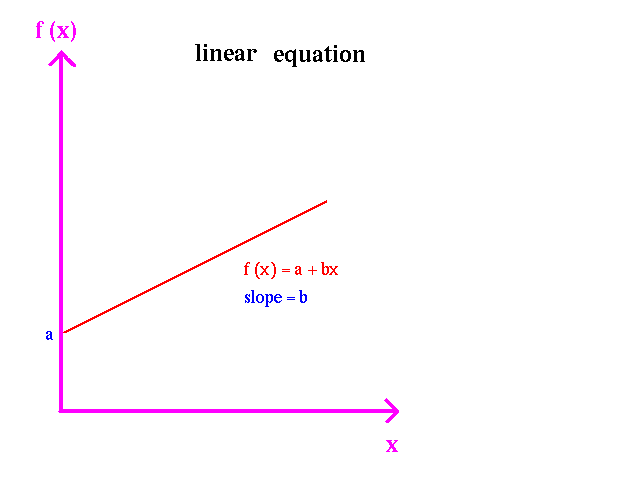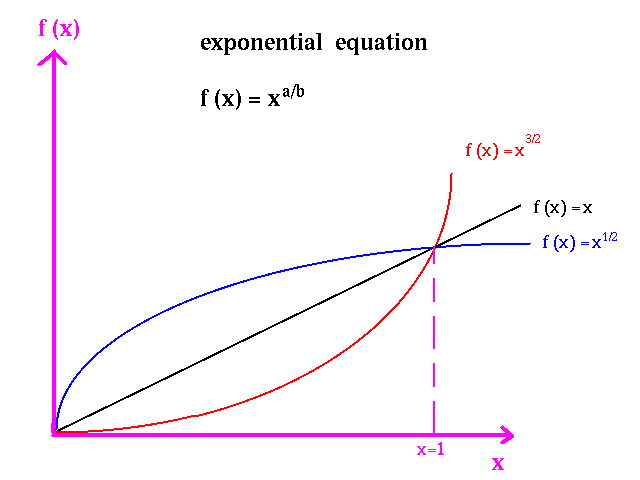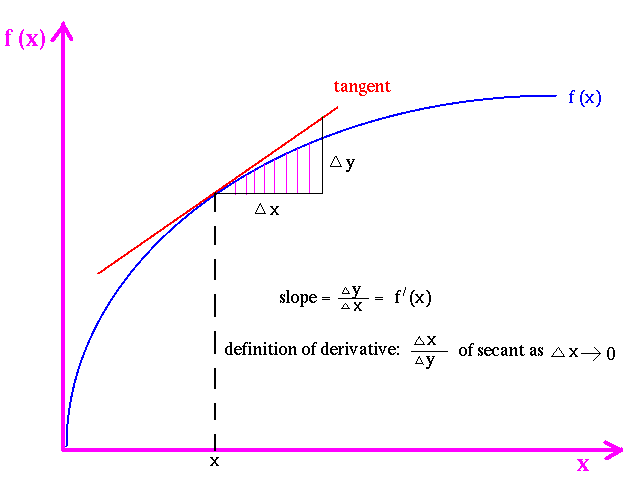## Math for Microeconomics

### Introduction

In this section we shall present the basic math tool to solve one variable optimization problems. In this course math is a tool to solve economics problems.

### Derivative

Function f: For any element x,  f(x) is an element in the range of f corresponding to element x in the domain. A function f can be represented as a graph {(x,y): y =f(x)} for all x in the domain of f.

The most common functions used in Economics are linear functions, functions to fractional exponents, and ln functions.
Linear: f(x) = a + bx
Fractional exponent: f(x) = xa/b
Ln f(x) = a + b ln(x)Derivative f'(x): The derivative of f at x, denoted as f'(x) or df/dx is defined as:
f'(x) = lim([f(x+h) - f(x)]/h) as h -> 0

The type of functions we will consider in this course will be differentiable at almost all points in their domains.

It is important to note that the derivative has a geometric interpretation. The derivative f'(x) is the slope of the tangent line to f at x.In taking derivatives we could go back to first principles and use the definition to derive them. It is much easier to use the rules derived from the definitions.

#### Derivative Rules

1. f(x) = xn f'(x) = n xn-1
2. f(x) = xa/b f'(x) = (a/b) x(a/b)-1
3. f(x) = ln x f'(x) = 1/x<

Examples:
1. f(x) = x f'(x) = x0 = 1
2. f(x) = x4 f'(x) = 4x3
3. f(x) = x2/3 f'(x) = (2/3)x-(1/3) = 2/[3x(1/3)]

There are three rules involving functions of functions which we will use repeatedly in the course.

1 Product Rule: Given functions g(x) and h(x) with f(x) = g(x)h(x):

f'(x) = g'(x)h(x) + g(x)h'(x)

2.Quotient Rule: Given functions g(x) and h(x) with f(x) = g(x)/h(x):

f'(x) = [g'(x)h(x) - g(x)h'(x)]/[h(x)]2

3.Composite Function Rule: Given functions g(x) and  y= h(x) with f(x) = g(h(x)) = g(y) What this means is that for a given x, y = h(x) is the input for g( ) :

f'(x) = g'(y)h'(x)
We could also write it as:
df/dx = (dg/dy)(dy/dx).

Examples:

1. f(x) = ln (x2)
_ f'(x) = (1/x2)2x = 2/x

2. f(x) = g(x)h(x) = (x2 - 3x)(2x3 - 1)
_ f'(x) = g'(x)h(x) + h'(x)g(x)
_____ = (2x - 3)(2x3 - 1) + (6x2)(x2 - 3x)
_____ = 10x4 - 24x3 - 2x + 3

3. f(x) = (3x2 + 2x + 1)2
_ f'(x) = 2(3x2 + 2x + 1)(6x + 2)

4. f(x) = g(x)/h(x) = x2/(2x - 1)
_ f'(x) = (g'(x)h(x) - h'(x)g(x))/(h(x))2
_____ = (2x(2x - 1) - 2x2)/(2x - 1)2
_____ = (2x2 - 2x)/(2x - 1)2

5. f(x) = g(x)/h(x) = (x2 - 3)/x2
_ f'(x) = (g'(x)h(x) - h'(x)g(x))/(h(x))2
_____ = (2x(x2) - 2x(x2 - 3))/(x2)2 = 6/x3

6. f(x) = g(x)h(x) = 2x2(3x4 - 2)
_ f'(x) = g'(x)h(x) + h'(x)g(x) = 4x(3x4 - 2) + 2x2(12x3)
_____ = 36x5 - 8x

7. f(x) = 3 ln(x2 - 1)3
_ f(x) = 3 ln(y);____ y = w3; ____w = x2 - 1
_ f'(x) = (3/y)3w2(2x)
_____ = (3 [3(x2 - 1)2 (2x)]/(x2 - 1)3
_____ = 18x/(x2 - 1)

8. f(x) = (x2 + 1)3(x3 - 1)2
__ f(x) = uv;____ u = w3; w = x2 + 1; v = z2; z = x3 - 1.
_ f'(x) = v (3w22x) + u(2z3x2)
_____ = 6(x3 - 1)2(x2 + 1)2x + 6(x2 + 1)3(x3 - 1)x2